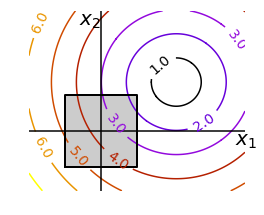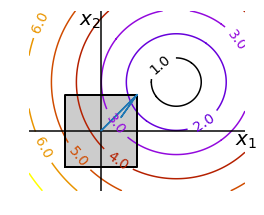# Scipy - Optimization¶

In :
import addutils.toc ; addutils.toc.js(ipy_notebook=True)

Out:
In :
import bokeh.plotting as bk
css_notebook()

Out:
In :
bk.output_notebook()


## 1 Brief Introduction to Optimization¶

### 1.1 Dimensionality of the problem¶

The scale of an optimization problem is pretty much set by the dimensionality of the problem, i.e. the number of scalar variables on which the search is performed.

### 1.2 Convex versus non-convex optimization¶

Convex functions can be optimized easily with gradient-descend algorithms. Non-convex functions optimization problems are much more difficult, expecially for higher dimensionality problems. This second class of optimization problems are not analyzed in this brief tutorial but are part of a specialised course.Convex functionNon Convex Function

### 1.3 Smooth and non-smooth problems¶

In a smooth problem the gradient is defined everywhere: this helps in speeding-up the optimization algorithms.Smooth functionNon Smooth Function

## 2 A Simpe Optimization Example¶

The scipy.optimize module provides useful algorithms for function minimization (scalar or multi-dimensional), curve fitting and root finding.

Let's find the minimum of this simple smooth non-convex function:

In :
import numpy as np

In :
from scipy import optimize
import numpy as np

def f(x):
return x**2 + 10*np.sin(x)

x = np.arange(-10, 10, 0.1)

fig = bk.figure()
fig.plot_height = 600
fig.plot_width = 600
fig.line(x, f(x))
bk.show(fig)


This function has a global minimum around -1.3 and a local minimum around 3.8.

The general and efficient way to find a minimum for this function is to conduct a gradient descent starting from a given initial point. The BFGS (quasi-Newton method of Broyden, Fletcher, Goldfarb, and Shanno) algorithm is a good way of doing this:

In :
optimize.fmin_bfgs(f, 0)

Optimization terminated successfully.
Current function value: -7.945823
Iterations: 5
Function evaluations: 18

Out:
array([-1.30644012])

A possible issue with this approach is that, if the function has local minima, the algorithm may find these local minima instead of the global minimum. scipy.optimize.anneal() provides an alternative, using simulated annealing. More efficient algorithms for different classes of global optimization problems exist, but this is out of the scope of scipy. Some useful packages for global optimization are OpenOpt, PyGMO and PyEvolve.

## 3 Finding the roots of a scalar function¶

To find a root, i.e. a point where f(x) = 0, of the function f above we can use for example scipy.optimize.fsolve():

In :
root = optimize.fsolve(f, 1)
root

Out:
array([0.])

Note that only one root is found. Inspecting the plot of f reveals that there is a second root around -2.5. We find the exact value of it by adjusting our initial guess:

In :
root2 = optimize.fsolve(f, -2.5)
root2

Out:
array([-2.47948183])

## 4 Curve Fitting¶

Suppose we have data sampled from f with some noise:

In :
xdata = np.linspace(-10, 10, num=20)
ydata = f(xdata) + np.random.randn(xdata.size)


Now if we know the functional form of the function from which the samples were drawn (x^2 + sin(x) in this case) but not the amplitudes of the terms, we can find those by least squares curve fitting. First we have to define the function to fit:

In :
def f2(x, a, b):
return a*x**2 + b*np.sin(x)


Then we can use scipy.optimize.curve_fit() to find a and b:

In :
guess = [2, 2]
params, params_covariance = optimize.curve_fit(f2, xdata, ydata, guess)

grid = (-10, 10, 0.1)
xmin_global = optimize.brute(f, (grid,))
xmin_local = optimize.fminbound(f, 0, 10)

fig = bk.figure(title=None)
fig.line(x, f(x), color='blue', line_width=2, legend="f(x)")
fig.line(x, f2(x, *params), color='red', line_width=2, line_dash=, legend="Curve fit result")

xmins = np.array([xmin_global, xmin_local])

fig.circle(xmins, f(xmins), size=9, color='green', legend="Minima")

roots = np.array([root, root2])

fig.triangle(roots, f(roots), size=9, color="black", legend="Roots")
fig.xaxis.axis_label = 'x'
fig.yaxis.axis_label = 'f(x)'
bk.show(fig)


## 5 Optimizing with Box-Bound Constraints¶

Box bounds correspond to limiting each of the individual parameters of the optimization. Note that some problems that are not originally written as box bounds can be rewritten as such be a change of variables.

• scipy.optimize.fminbound() for 1D-optimization
• scipy.optimize.fmin_l_bfgs_b() a quasi-Newton method with bound constraints
In :
import warnings
warnings.filterwarnings('ignore', category=UnicodeWarning)

In :
%matplotlib inline
import matplotlib.pyplot as plt

x, y = np.mgrid[-2.9:5.8:.05, -2.5:5:.05]
x = x.T
y = y.T

for i in (1, 2):
# Create 2 figure: only the second one will have the optimization
# path
plt.figure(i, figsize=(3, 2.5))
plt.clf()
plt.axes([0, 0, 1, 1])

contours = plt.contour(np.sqrt((x - 3)**2 + (y - 2)**2),
extent=[-3, 6, -2.5, 5], cmap=plt.cm.gnuplot)
plt.clabel(contours, inline=1, fmt='%1.1f', fontsize=14)

plt.plot([-1.5, -1.5,  1.5,  1.5, -1.5],
[-1.5,  1.5,  1.5, -1.5, -1.5], 'k', linewidth=2)
plt.fill_between([ -1.5,  1.5],
[ -1.5, -1.5],
[  1.5,  1.5],
color='.8')
plt.axvline(0, color='k')
plt.axhline(0, color='k')

plt.text(-.9, 4.4, '$x_2$', size=20)
plt.text(5.6, -.6, '$x_1$', size=20)
plt.axis('equal')
plt.axis('off')

# And now plot the optimization path
accumulator = list()

def f(x):
# Store the list of function calls
accumulator.append(x)
return np.sqrt((x - 3)**2 + (x - 2)**2)

# We don't use the gradient, as with the gradient, L-BFGS is too fast,
# and finds the optimum without showing us a pretty path
def f_prime(x):
r = np.sqrt((x - 3)**2 + (x - 2)**2)
return np.array(((x - 3)/r, (x - 2)/r))


[<matplotlib.lines.Line2D at 0x7fd7a482d470>]